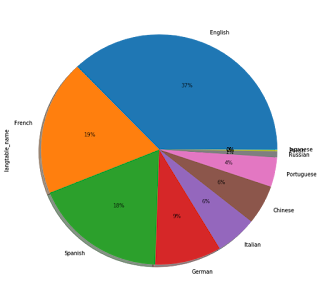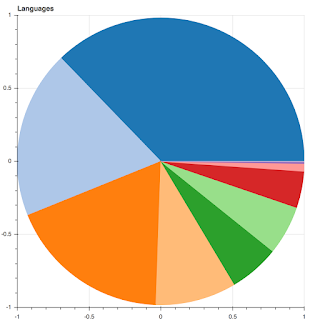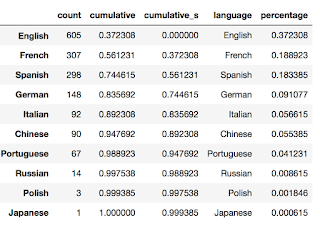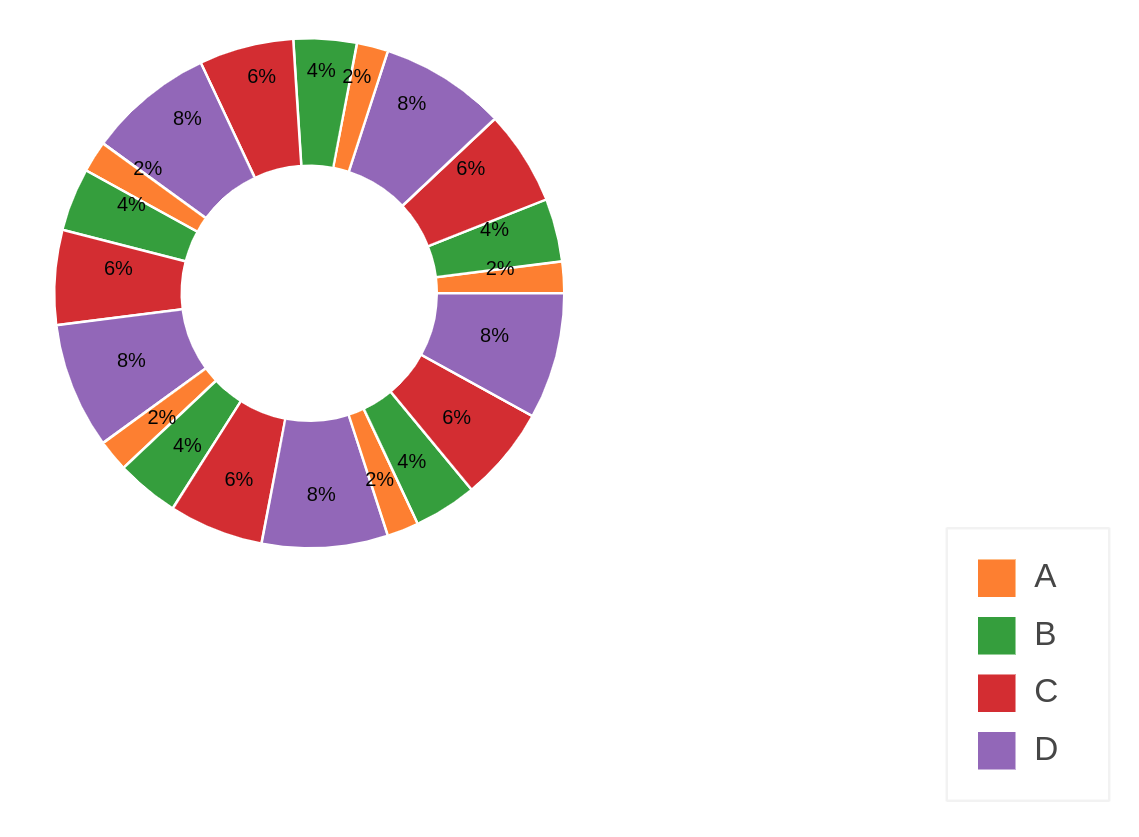# Wedge Pie Chart labels

Dear all,

I am working on a set of data where I have a list of languages and a number of users per language. Since I want to move all my works to Bokeh I was trying to create a pie chart which I had created before using Pandas/Matplotlib. The original chart is the following:After working a bit with the wedge I was able to recreate the pie chart but I still can’t figure out how to add the labels on the chart, either with the hover (preferred) or also directly onto the chart.The data comes from this pandas.DataFrame which was converted to a ColumnDataSource:And the code used is the following:

starts = [p2np.pi for p in source.data[‘cumulative_s’]]
ends = [p2np.pi for p in source.data[‘cumulative’]]

p = figure(x_range=(-1,1), y_range=(-1,1),tools=[“save”], title=“Languages”)

p.wedge(x=0, y=0, radius=1, start_angle=starts, end_angle=ends, color=Category20[complete.shape])

``

Dear all,

I solved it on my own; I had to move everything inside the ColumnDataSource, included the start_angle, end_angle and colours.

This is the final code that worked (with hovertool):

hover = HoverTool(tooltips=[(“language”,"@label"),

(“percentage”, “@percentage{%0.2f}”)

])

p = figure(x_range=(-1,1), y_range=(-1,1),tools=[“save”, hover], title=“Languages”)

p.wedge(x=0, y=0, radius=1, start_angle=“cumulative_s”, end_angle=“cumulative”, color=“colour”, source=source)

``

Thank you, I love Bokeh and I wish I’ll be able to contribute in a near future.

···

On Wednesday, 3 January 2018 17:29:48 UTC+1, Filippo B wrote:

Dear all,

I am working on a set of data where I have a list of languages and a number of users per language. Since I want to move all my works to Bokeh I was trying to create a pie chart which I had created before using Pandas/Matplotlib. The original chart is the following:After working a bit with the wedge I was able to recreate the pie chart but I still can’t figure out how to add the labels on the chart, either with the hover (preferred) or also directly onto the chart.The data comes from this pandas.DataFrame which was converted to a ColumnDataSource:And the code used is the following:

starts = [p2np.pi for p in source.data[‘cumulative_s’]]
ends = [p2np.pi for p in source.data[‘cumulative’]]

p = figure(x_range=(-1,1), y_range=(-1,1),tools=[“save”], title=“Languages”)

p.wedge(x=0, y=0, radius=1, start_angle=starts, end_angle=ends, color=Category20[complete.shape])

``

Thank you for the kind words, I'm glad you figured things out and look forward to any contributions you can make!

Bryan

···

On Jan 3, 2018, at 12:00, Filippo B <[email protected]> wrote:

Dear all,

I solved it on my own; I had to move everything inside the ColumnDataSource, included the start_angle, end_angle and colours.

This is the final code that worked (with hovertool):

hover = HoverTool(tooltips=[("language","@label"),
("percentage", "@percentage{%0.2f}")
])

p = figure(x_range=(-1,1), y_range=(-1,1),tools=["save", hover], title="Languages")
p.wedge(x=0, y=0, radius=1, start_angle="cumulative_s", end_angle="cumulative", color="colour", source=source)

Thank you, I love Bokeh and I wish I'll be able to contribute in a near future.

On Wednesday, 3 January 2018 17:29:48 UTC+1, Filippo B wrote:
Dear all,

I am working on a set of data where I have a list of languages and a number of users per language. Since I want to move all my works to Bokeh I was trying to create a pie chart which I had created before using Pandas/Matplotlib. The original chart is the following:

After working a bit with the wedge I was able to recreate the pie chart but I still can't figure out how to add the labels on the chart, either with the hover (preferred) or also directly onto the chart.

The data comes from this pandas.DataFrame which was converted to a ColumnDataSource:

And the code used is the following:

starts = [p*2*np.pi for p in source.data['cumulative_s']]
ends = [p*2*np.pi for p in source.data['cumulative']]

p = figure(x_range=(-1,1), y_range=(-1,1),tools=["save"], title="Languages")

p.wedge(x=0, y=0, radius=1, start_angle=starts, end_angle=ends, color=Category20[complete.shape])

--
You received this message because you are subscribed to the Google Groups "Bokeh Discussion - Public" group.
To unsubscribe from this group and stop receiving emails from it, send an email to [email protected].
To post to this group, send email to [email protected].

I didn’t get how you solved the issue plz could you help more I want to show the labels inside the circle without hover over each part?

···

Le mercredi 3 janvier 2018 19:00:41 UTC+1, Filippo B a écrit :

Dear all,

I solved it on my own; I had to move everything inside the ColumnDataSource, included the start_angle, end_angle and colours.

This is the final code that worked (with hovertool):

hover = HoverTool(tooltips=[(“language”,"@label"),

(“percentage”, “@percentage{%0.2f}”)

])

p = figure(x_range=(-1,1), y_range=(-1,1),tools=[“save”, hover], title=“Languages”)

p.wedge(x=0, y=0, radius=1, start_angle=“cumulative_s”, end_angle=“cumulative”, color=“colour”, source=source)

``

Thank you, I love Bokeh and I wish I’ll be able to contribute in a near future.

On Wednesday, 3 January 2018 17:29:48 UTC+1, Filippo B wrote:

Dear all,

I am working on a set of data where I have a list of languages and a number of users per language. Since I want to move all my works to Bokeh I was trying to create a pie chart which I had created before using Pandas/Matplotlib. The original chart is the following:After working a bit with the wedge I was able to recreate the pie chart but I still can’t figure out how to add the labels on the chart, either with the hover (preferred) or also directly onto the chart.The data comes from this pandas.DataFrame which was converted to a ColumnDataSource:And the code used is the following:

starts = [p2np.pi for p in source.data[‘cumulative_s’]]
ends = [p2np.pi for p in source.data[‘cumulative’]]

p = figure(x_range=(-1,1), y_range=(-1,1),tools=[“save”], title=“Languages”)

p.wedge(x=0, y=0, radius=1, start_angle=starts, end_angle=ends, color=Category20[complete.shape])

``

Could you please kindly post your full code for it? I’d highly appreciate it.

Dear all,

I solved it on my own; I had to move everything inside the ColumnDataSource, included the start_angle, end_angle and colours.

This is the final code that worked (with hovertool):

hover = HoverTool(tooltips=[(“language”,"@label"),

(“percentage”, “@percentage{%0.2f}”)

])

p = figure(x_range=(-1,1), y_range=(-1,1),tools=[“save”, hover], title=“Languages”)

p.wedge(x=0, y=0, radius=1, start_angle=“cumulative_s”, end_angle=“cumulative”, color=“colour”, source=source)

``

Thank you, I love Bokeh and I wish I’ll be able to contribute in a near future.

Dear all,

I am working on a set of data where I have a list of languages and a number of users per language. Since I want to move all my works to Bokeh I was trying to create a pie chart which I had created before using Pandas/Matplotlib. The original chart is the following:After working a bit with the wedge I was able to recreate the pie chart but I still can’t figure out how to add the labels on the chart, either with the hover (preferred) or also directly onto the chart.The data comes from this pandas.DataFrame which was converted to a ColumnDataSource:And the code used is the following:

starts = [p2np.pi for p in source.data[‘cumulative_s’]]
ends = [p2np.pi for p in source.data[‘cumulative’]]

p = figure(x_range=(-1,1), y_range=(-1,1),tools=[“save”], title=“Languages”)

p.wedge(x=0, y=0, radius=1, start_angle=starts, end_angle=ends, color=Category20[complete.shape])

``

*This e-mail and any
attachments may contain confidential information and is intended for use solely
by the addressee(s). If you are not the
intended recipient of this e-mail, please be aware that any dissemination,
distribution, copying, or other use of the e-mail in whole or in part, is
strictly prohibited. If you have
received this e-mail in error, please notify the sender and permanently delete
the original and all copies of the e-mail, attachments, and any printouts. *****

···

On Wednesday, January 3, 2018 at 1:00:41 PM UTC-5, Filippo B wrote:

On Wednesday, 3 January 2018 17:29:48 UTC+1, Filippo B wrote:

Hi,

I don’t have the original chart anymore but I’ll post you the full code of a newer version of it. This is designed to start at pi/2 clockwise, which is the reference style for a pie chart. When I originally designed the chart, there was a problem with creating pie charts clockwise, so what I did was creating a chart anticlockwise starting from the smaller bin, which has the practical effect of creating it clockwise starting from the biggest bin. Please note that this code still works in Bokeh 1.x but it might not be the most elegant way to do it (this code outputs to the Jupyter Notebook but if you want an HTML just uncomment the corresponding line at the end):

import pandas as pd

import numpy as np

from bokeh.plotting import figure, output_file, ColumnDataSource, save, output_notebook, show

from bokeh.models import HoverTool

from bokeh.palettes import Category20

# example source data

languages = pd.Series([‘Japanese’, ‘Polish’, ‘Russian’, ‘Portuguese’])

counts = pd.Series([12, 45, 67, 98])

# calculate the percentages of each language

percentage = counts/counts.sum()

# calculate the cumulative distribution of percentages, necessary for the Bokeh chart

cumulative = counts.cumsum()/counts.sum()

#creating the series starting with zero and shifted of one position compared to cumulative

# necessary for the Bokeh chart

cumulative_s = cumulative.copy()

cumulative_s = 0

cumulative_s[1:] = cumulative[:-1]

# + 0.5*np.pi means letting the bigger language start from 12:00 and anticlockwise

starts = cumulative_s2np.pi + 0.5*np.pi

ends = cumulative2np.pi + 0.5*np.pi

colour = pd.Series(Category20[cumulative.shape], index=cumulative.index)

#creating a dataframe out of the information created so far, and then converting it to a ColumnDataSource

complete = pd.DataFrame({“count”: counts,

“percentage”: percentage,

“cumulative_s”: starts,

“cumulative”: ends,

“label”: languages,

“colour”: colour,

})

source = ColumnDataSource(data=complete)

# hover tool definition

hover = HoverTool(tooltips=[(“language”,"@label"),

(“percentage”, “@percentage{%0.2f}”)

])

# chart definition

p.wedge(x=0, y=0, radius=0.9, start_angle=“cumulative_s”, end_angle=“cumulative”, color=“colour”, source=source)

p.toolbar.logo = None

p.toolbar_location = None

p.xgrid.visible = False

p.ygrid.visible = False

p.axis.visible = False

#Output to static HTML file

#output_file(“test.html”)

#Output to Jupyter Notebook

show§

``

···

On Tuesday, 22 January 2019 16:37:02 UTC+1, hk331 wrote:

Could you please kindly post your full code for it? I’d highly appreciate it.

On Wednesday, January 3, 2018 at 1:00:41 PM UTC-5, Filippo B wrote:

Dear all,

I solved it on my own; I had to move everything inside the ColumnDataSource, included the start_angle, end_angle and colours.

This is the final code that worked (with hovertool):

hover = HoverTool(tooltips=[(“language”,"@label"),

(“percentage”, “@percentage{%0.2f}”)

])

p = figure(x_range=(-1,1), y_range=(-1,1),tools=[“save”, hover], title=“Languages”)

p.wedge(x=0, y=0, radius=1, start_angle=“cumulative_s”, end_angle=“cumulative”, color=“colour”, source=source)

``

Thank you, I love Bokeh and I wish I’ll be able to contribute in a near future.

On Wednesday, 3 January 2018 17:29:48 UTC+1, Filippo B wrote:

Dear all,

I am working on a set of data where I have a list of languages and a number of users per language. Since I want to move all my works to Bokeh I was trying to create a pie chart which I had created before using Pandas/Matplotlib. The original chart is the following:After working a bit with the wedge I was able to recreate the pie chart but I still can’t figure out how to add the labels on the chart, either with the hover (preferred) or also directly onto the chart.The data comes from this pandas.DataFrame which was converted to a ColumnDataSource:And the code used is the following:

starts = [p2np.pi for p in source.data[‘cumulative_s’]]
ends = [p2np.pi for p in source.data[‘cumulative’]]

p = figure(x_range=(-1,1), y_range=(-1,1),tools=[“save”], title=“Languages”)

p.wedge(x=0, y=0, radius=1, start_angle=starts, end_angle=ends, color=Category20[complete.shape])

``

*This e-mail and any
attachments may contain confidential information and is intended for use solely
by the addressee(s). If you are not the
intended recipient of this e-mail, please be aware that any dissemination,
distribution, copying, or other use of the e-mail in whole or in part, is
strictly prohibited. If you have
received this e-mail in error, please notify the sender and permanently delete
the original and all copies of the e-mail, attachments, and any printouts. *****

Thanks a lot Filippo but, weirdly enough!, your code does not work for me. I want percentage TEXTS on the Pie chart. Please take a look at my code; I used some Cos, Sin trick to do that; however, when the chart width changes the texts might get scattered for some reason! Mathematically it should be always correct, I guess it might be because of Bokeh inner dimension which I don’t know how exactly they work.

``````
from math import pi

from bokeh.palettes import Category10

from bokeh.models.sources import ColumnDataSource

from bokeh.plotting import figure

from bokeh.transform import cumsum

from bokeh.models import Legend, HoverTool, LabelSet, LegendItem

from bokeh.io import output_notebook, show, push_notebook

import numpy as np

output_notebook()

value = [10,20,30,40]*5

data = {'name': ["A", "B","C","D"]*5,

'value': value,

'angle': [v/sum(value)*2*pi for v in value],

'cumulative_angle':[(sum(value[0:i+1])- (item/2))/sum(value)*2*pi for i,item in enumerate(value)],

'percentage': [d/sum(value)*100 for d in value],

'color': Category10[1:]*5}

data['label'] = ["{:.0f}%".format(p) for p in data['percentage']]

data['cos'] = np.cos(data['cumulative_angle'])*0.3

data['sin'] = np.sin(data['cumulative_angle'])*0.3

source = ColumnDataSource(data=data)

TOOLTIPS = [

("Value", "@value"),

("Percentage", "@percentage{0.2f}%")

]

p = figure(plot_height=600,plot_width=600,x_range=(-1,1), y_range=(-1,1), tools='hover',

tooltips=TOOLTIPS, title="My Donut Chart", toolbar_location=None)

start_angle=cumsum('angle', include_zero=True), end_angle=cumsum('angle'),

line_color="white", fill_color='color', fill_alpha=1, source=source)

legend = Legend(items=[LegendItem(label=dict(field="name"), renderers=[r])], location=(0, 80))

labels = LabelSet(x='cos', y='sin', text="label", y_offset=0,

text_font_size="6pt", text_color="black",

source=source, text_align='center')

p.axis.axis_label=None

p.axis.visible=False

p.grid.grid_line_color = None

p.outline_line_color = None

show(p)

``````

it results in:any improvement, idea etc. is appreciated! also, I only get the hover when I run YOUR code. Thanks

Hi,

I don’t have the original chart anymore but I’ll post you the full code of a newer version of it. This is designed to start at pi/2 clockwise, which is the reference style for a pie chart. When I originally designed the chart, there was a problem with creating pie charts clockwise, so what I did was creating a chart anticlockwise starting from the smaller bin, which has the practical effect of creating it clockwise starting from the biggest bin. Please note that this code still works in Bokeh 1.x but it might not be the most elegant way to do it (this code outputs to the Jupyter Notebook but if you want an HTML just uncomment the corresponding line at the end):

import pandas as pd

import numpy as np

from bokeh.plotting import figure, output_file, ColumnDataSource, save, output_notebook, show

from bokeh.models import HoverTool

from bokeh.palettes import Category20

# example source data

languages = pd.Series([‘Japanese’, ‘Polish’, ‘Russian’, ‘Portuguese’])

counts = pd.Series([12, 45, 67, 98])

# calculate the percentages of each language

percentage = counts/counts.sum()

# calculate the cumulative distribution of percentages, necessary for the Bokeh chart

cumulative = counts.cumsum()/counts.sum()

#creating the series starting with zero and shifted of one position compared to cumulative

# necessary for the Bokeh chart

cumulative_s = cumulative.copy()

cumulative_s = 0

cumulative_s[1:] = cumulative[:-1]

# + 0.5*np.pi means letting the bigger language start from 12:00 and anticlockwise

starts = cumulative_s2np.pi + 0.5*np.pi

ends = cumulative2np.pi + 0.5*np.pi

colour = pd.Series(Category20[cumulative.shape], index=cumulative.index)

#creating a dataframe out of the information created so far, and then converting it to a ColumnDataSource

complete = pd.DataFrame({“count”: counts,

“percentage”: percentage,

“cumulative_s”: starts,

“cumulative”: ends,

“label”: languages,

“colour”: colour,

})

source = ColumnDataSource(data=complete)

# hover tool definition

hover = HoverTool(tooltips=[(“language”,"@label"),

(“percentage”, “@percentage{%0.2f}”)

])

# chart definition

p.wedge(x=0, y=0, radius=0.9, start_angle=“cumulative_s”, end_angle=“cumulative”, color=“colour”, source=source)

p.toolbar.logo = None

p.toolbar_location = None

p.xgrid.visible = False

p.ygrid.visible = False

p.axis.visible = False

#Output to static HTML file

#output_file(“test.html”)

#Output to Jupyter Notebook

show§

``

Could you please kindly post your full code for it? I’d highly appreciate it.

Dear all,

I solved it on my own; I had to move everything inside the ColumnDataSource, included the start_angle, end_angle and colours.

This is the final code that worked (with hovertool):

hover = HoverTool(tooltips=[(“language”,"@label"),

(“percentage”, “@percentage{%0.2f}”)

])

p = figure(x_range=(-1,1), y_range=(-1,1),tools=[“save”, hover], title=“Languages”)

p.wedge(x=0, y=0, radius=1, start_angle=“cumulative_s”, end_angle=“cumulative”, color=“colour”, source=source)

``

Thank you, I love Bokeh and I wish I’ll be able to contribute in a near future.

Dear all,

I am working on a set of data where I have a list of languages and a number of users per language. Since I want to move all my works to Bokeh I was trying to create a pie chart which I had created before using Pandas/Matplotlib. The original chart is the following:After working a bit with the wedge I was able to recreate the pie chart but I still can’t figure out how to add the labels on the chart, either with the hover (preferred) or also directly onto the chart.The data comes from this pandas.DataFrame which was converted to a ColumnDataSource:And the code used is the following:

starts = [p2np.pi for p in source.data[‘cumulative_s’]]
ends = [p2np.pi for p in source.data[‘cumulative’]]

p = figure(x_range=(-1,1), y_range=(-1,1),tools=[“save”], title=“Languages”)

p.wedge(x=0, y=0, radius=1, start_angle=starts, end_angle=ends, color=Category20[complete.shape])

``

*This e-mail and any
attachments may contain confidential information and is intended for use solely
by the addressee(s). If you are not the
intended recipient of this e-mail, please be aware that any dissemination,
distribution, copying, or other use of the e-mail in whole or in part, is
strictly prohibited. If you have
received this e-mail in error, please notify the sender and permanently delete
the original and all copies of the e-mail, attachments, and any printouts. *****

*This e-mail and any
attachments may contain confidential information and is intended for use solely
by the addressee(s). If you are not the
intended recipient of this e-mail, please be aware that any dissemination,
distribution, copying, or other use of the e-mail in whole or in part, is
strictly prohibited. If you have
received this e-mail in error, please notify the sender and permanently delete
the original and all copies of the e-mail, attachments, and any printouts. *****

···

On Wednesday, January 23, 2019 at 3:59:09 AM UTC-5, Filippo B wrote:

On Tuesday, 22 January 2019 16:37:02 UTC+1, hk331 wrote:

On Wednesday, January 3, 2018 at 1:00:41 PM UTC-5, Filippo B wrote:

On Wednesday, 3 January 2018 17:29:48 UTC+1, Filippo B wrote:

Hi, you most likely want to set match_aspect to True for the figure so the ranges are adjusted to ensure there is always a 1-1 data space to pixel aspect ratio.

Thanks,

Bryan

···

On Jan 23, 2019, at 07:03, [email protected] wrote:

Thanks a lot Filippo but, weirdly enough!, your code does not work for me. I want percentage TEXTS on the Pie chart. Please take a look at my code; I used some Cos, Sin trick to do that; however, when the chart width changes the texts might get scattered for some reason! Mathematically it should be always correct, I guess it might be because of Bokeh inner dimension which I don’t know how exactly they work.

from math import pi

from bokeh.palettes import Category10

from bokeh.models.sources import ColumnDataSource

from bokeh.plotting import figure

from bokeh.transform import cumsum

from bokeh.models import Legend, HoverTool, LabelSet, LegendItem

from bokeh.io import output_notebook, show, push_notebook

import numpy as np

output_notebook()

value = [10,20,30,40]*5

data = {‘name’: [“A”, “B”,“C”,“D”]*5,

‘value’: value,

‘angle’: [v/sum(value)2pi for v in value],

‘cumulative_angle’:[(sum(value[0:i+1])- (item/2))/sum(value)2pi for i,item in enumerate(value)],

‘percentage’: [d/sum(value)*100 for d in value],

‘color’: Category10[1:]*5}

data[‘label’] = ["{:.0f}%".format§ for p in data[‘percentage’]]

data[‘cos’] = np.cos(data[‘cumulative_angle’])*0.3

data[‘sin’] = np.sin(data[‘cumulative_angle’])*0.3

source = ColumnDataSource(data=data)

TOOLTIPS = [

(“Value”, “@value”),

(“Percentage”, “@percentage{0.2f}%”)

]

p = figure(plot_height=600,plot_width=600,x_range=(-1,1), y_range=(-1,1), tools=‘hover’,

tooltips=TOOLTIPS, title=“My Donut Chart”, toolbar_location=None)

start_angle=cumsum(‘angle’, include_zero=True), end_angle=cumsum(‘angle’),

line_color=“white”, fill_color=‘color’, fill_alpha=1, source=source)

legend = Legend(items=[LegendItem(label=dict(field=“name”), renderers=[r])], location=(0, 80))

labels = LabelSet(x=‘cos’, y=‘sin’, text=“label”, y_offset=0,

text_font_size=“6pt”, text_color=“black”,

source=source, text_align=‘center’)

p.axis.axis_label=None

p.axis.visible=False

p.grid.grid_line_color = None

p.outline_line_color = None

show§

it results in:

<Screenshot from 2019-01-22 14-07-07.png>

any improvement, idea etc. is appreciated! also, I only get the hover when I run YOUR code. Thanks

On Wednesday, January 23, 2019 at 3:59:09 AM UTC-5, Filippo B wrote:

Hi,

I don’t have the original chart anymore but I’ll post you the full code of a newer version of it. This is designed to start at pi/2 clockwise, which is the reference style for a pie chart. When I originally designed the chart, there was a problem with creating pie charts clockwise, so what I did was creating a chart anticlockwise starting from the smaller bin, which has the practical effect of creating it clockwise starting from the biggest bin. Please note that this code still works in Bokeh 1.x but it might not be the most elegant way to do it (this code outputs to the Jupyter Notebook but if you want an HTML just uncomment the corresponding line at the end):

import pandas as pd

import numpy as np

from bokeh.plotting import figure, output_file, ColumnDataSource, save, output_notebook, show

from bokeh.models import HoverTool

from bokeh.palettes import Category20

# example source data

languages = pd.Series([‘Japanese’, ‘Polish’, ‘Russian’, ‘Portuguese’])

counts = pd.Series([12, 45, 67, 98])

# calculate the percentages of each language

percentage = counts/counts.sum()

# calculate the cumulative distribution of percentages, necessary for the Bokeh chart

cumulative = counts.cumsum()/counts.sum()

#creating the series starting with zero and shifted of one position compared to cumulative

# necessary for the Bokeh chart

cumulative_s = cumulative.copy()

cumulative_s = 0

cumulative_s[1:] = cumulative[:-1]

# + 0.5*np.pi means letting the bigger language start from 12:00 and anticlockwise

starts = cumulative_s2np.pi + 0.5*np.pi

ends = cumulative2np.pi + 0.5*np.pi

colour = pd.Series(Category20[cumulative.shape], index=cumulative.index)

#creating a dataframe out of the information created so far, and then converting it to a ColumnDataSource

complete = pd.DataFrame({“count”: counts,

“percentage”: percentage,

“cumulative_s”: starts,

“cumulative”: ends,

“label”: languages,

“colour”: colour,

})

source = ColumnDataSource(data=complete)

# hover tool definition

hover = HoverTool(tooltips=[(“language”,"@label"),

(“percentage”, “@percentage{%0.2f}”)

])

# chart definition

p.wedge(x=0, y=0, radius=0.9, start_angle=“cumulative_s”, end_angle=“cumulative”, color=“colour”, source=source)

p.toolbar.logo = None

p.toolbar_location = None

p.xgrid.visible = False

p.ygrid.visible = False

p.axis.visible = False

#Output to static HTML file

#output_file(“test.html”)

#Output to Jupyter Notebook

show§

``

On Tuesday, 22 January 2019 16:37:02 UTC+1, hk331 wrote:

Could you please kindly post your full code for it? I’d highly appreciate it.

On Wednesday, January 3, 2018 at 1:00:41 PM UTC-5, Filippo B wrote:

Dear all,

I solved it on my own; I had to move everything inside the ColumnDataSource, included the start_angle, end_angle and colours.

This is the final code that worked (with hovertool):

hover = HoverTool(tooltips=[(“language”,"@label"),

(“percentage”, “@percentage{%0.2f}”)

])

p = figure(x_range=(-1,1), y_range=(-1,1),tools=[“save”, hover], title=“Languages”)

p.wedge(x=0, y=0, radius=1, start_angle=“cumulative_s”, end_angle=“cumulative”, color=“colour”, source=source)

``

Thank you, I love Bokeh and I wish I’ll be able to contribute in a near future.

On Wednesday, 3 January 2018 17:29:48 UTC+1, Filippo B wrote:

Dear all,

I am working on a set of data where I have a list of languages and a number of users per language. Since I want to move all my works to Bokeh I was trying to create a pie chart which I had created before using Pandas/Matplotlib. The original chart is the following:After working a bit with the wedge I was able to recreate the pie chart but I still can’t figure out how to add the labels on the chart, either with the hover (preferred) or also directly onto the chart.The data comes from this pandas.DataFrame which was converted to a ColumnDataSource:And the code used is the following:

starts = [p2np.pi for p in source.data[‘cumulative_s’]]
ends = [p2np.pi for p in source.data[‘cumulative’]]

p = figure(x_range=(-1,1), y_range=(-1,1),tools=[“save”], title=“Languages”)

p.wedge(x=0, y=0, radius=1, start_angle=starts, end_angle=ends, color=Category20[complete.shape])

``

*This e-mail and any
attachments may contain confidential information and is intended for use solely
by the addressee(s). If you are not the
intended recipient of this e-mail, please be aware that any dissemination,
distribution, copying, or other use of the e-mail in whole or in part, is
strictly prohibited. If you have
received this e-mail in error, please notify the sender and permanently delete
the original and all copies of the e-mail, attachments, and any printouts. *****

*This e-mail and any
attachments may contain confidential information and is intended for use solely
by the addressee(s). If you are not the
intended recipient of this e-mail, please be aware that any dissemination,
distribution, copying, or other use of the e-mail in whole or in part, is
strictly prohibited. If you have
received this e-mail in error, please notify the sender and permanently delete
the original and all copies of the e-mail, attachments, and any printouts. *****

You received this message because you are subscribed to the Google Groups “Bokeh Discussion - Public” group.

To unsubscribe from this group and stop receiving emails from it, send an email to [email protected].

To post to this group, send email to [email protected].